# Jina: Now with Google Colab!Susana Guzmán

We all know how Google Colab can make things easier when trying new code, so we're super happy that after the latest Google Colab update, it can now run with Python 3.7, which is just what we need with Jina.And how we do that? Super easyyyy, no fuss needed, let's get to it!

## Just tell me how!

This is really straightforward, in this example we'll index some data for you to see how to run it with Google Colab, so you will see how to:

1. Install Jina
2. Import Jina Flow
3. Create a Flow

### 1. Install Jina

The first thing is to create a notebook in Google Colab. You can do that here and install Jina via `pip` with:

``````!pip install jina

``````

And you should have something like this: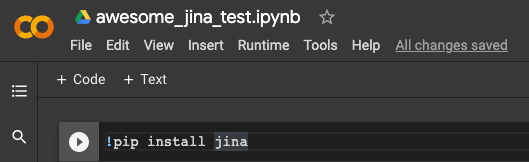### 2. Import Jina Flow

Then we need to import our Flow:

``````from jina import Flow

``````

### 3. Create a Flow

Now that we have Jina installed and we imported our Flow we can start using it. This is business as usual:

``````my_fluffy_flow = Flow()

``````

But let's try to make it a bit more interesting by adding two Pods, one for the Encoder and one for the Indexer:

``````my_fluffy_flow = Flow().add(name='encoder').add(name='indexer')

``````

So we should have something like this: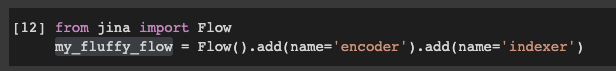Now, this is a simple example, but if you do more fancy stuff, you might want to check how your Flow is looking, so you can do that simply by referring to the name of your Flow, for example: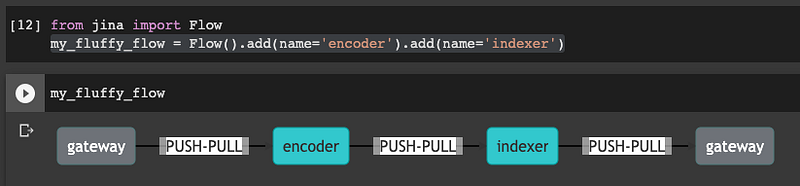There you can see we indeed have two Pods one after the other.

Okay, so we have our Flow ready with two Pods, now it's time to index some simple data. This is as simple as: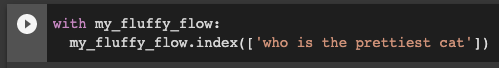And you are done! If you run this you will see that your indexing will start: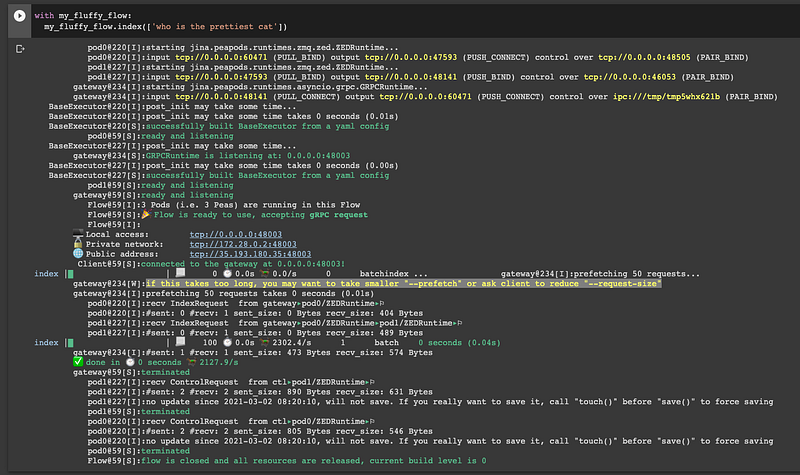This was a very simple demonstration of what you can do, but because Google Colab is so interactive, it makes it a very powerful tool to run any of the ideas you might have. If you want to run this example this is all you need:

``````!pip install jina                                                           #Install Jina

from jina import Flow                                                       #Import Flow
my_fluffy_flow = Flow()                                                     #Create Flow